Q.1) Convert:
₹ 15.5 = ___ paise.
a) 15500 paise
b) 1550 paise

Q.2) Convert:
3760 paise = ₹ _____
a) ₹ 376
b) ₹ 37.6

Q.3) Find the total amount.
2 notes of ₹ 500
4 notes of ₹ 100
3 notes of ₹ 50
5 notes of ₹ 10

a) ₹ 1600
b) ₹ 1450
c) ₹ 1700

₹ 289.24 and ₹ 367.27
a) ₹ 656.51
b) ₹ 654.11
c) ₹ 660.31

Q.5) Subtract the following:
₹ 513.67 and ₹ 238.15
a) ₹ 279.88
b) ₹ 271.16
c) ₹ 275.52

Q.6) If the cost of one ice-cream is ₹ 13.28, find the cost of 3 ice-creams ?
a) ₹ 39.84
b) ₹ 37.34
c) ₹ 42.09

Q.7) If the cost of 6 pens is ₹ 144,  find the cost of one pen?
a) ₹ 30
b) ₹ 24
c) ₹ 34

### Money Worksheets Grade 4 Explanations

Q.1) Explanation –

As we know that,
₹ 1 = 100 paise
₹ 15.5 = ( 15.5 x 100 ) paise
₹ 15.5 = 1550 paise

Correct Answer – b) 1550 paise

Q.2) Explanation – Money Worksheets Grade 4

As we know that, ₹ 1 = 100 paise
or 100 paise = ₹ 1
then,
1 paise = ₹ 1/100
To find 3760 paise in ₹ . We have to multiply both sides by 3760
( 3760 x 1 ) paise = ₹ ( 3760 x 1/100)
3760 paise = ₹ 37.6

Correct Answer – b) ₹ 37.6

Q.3) Explanation – Money Worksheets Grade 4

2 notes of ₹ 500 = ₹ ( 2 x 500 ) = ₹ 1000
4 notes of ₹ 100 = ₹ ( 4 x 100 ) = ₹ 400
3 notes of ₹ 50 = ₹ ( 3 x 50 ) = ₹ 150
5 notes of ₹ 10 = ₹ ( 5 x 10 ) = ₹ 50
Total amount = ₹ 1000 + ₹ 400 + ₹ 150 + ₹ 50
Total amount = ₹ 1600

Correct Answer – a) ₹ 1600

Q.4) Explanation – Money Worksheets Grade 4

Step I: Write Rupees ( ₹ ) and Paise ( p ) aligned in the rupees and paise columns.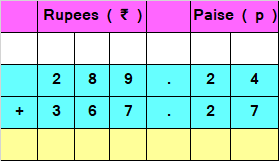Step II: Add Rupees ( ₹ ) and Paise ( p ) as we add numbers in Addition.

We would add the numbers in a normal way, as we do addition, starting from the right side, i.e paise side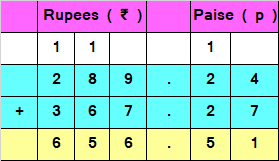Hence, the sum of given money is ₹ 656.51

Correct Answer – a) ₹ 656.51

Q.5) Explanation – Money Worksheets Grade 4

Step I: Write Rupees ( ₹ ) and Paise ( p ) aligned in the rupees and paise columns.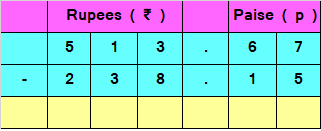Step II: Subtract Rupees ( ₹ ) and Paise ( p ) as we subtract numbers in Subtraction.

We would subtract the numbers in a normal way, as we do in subtraction, starting from the right side, i.e paise side.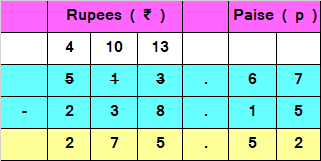Hence, the difference of given money is ₹ 275.52

Correct Answer – c) ₹ 275.52

Q.6) Explanation – Money Worksheets Grade 4

Here, we have to find the cost of 3 ice-creams when cost of 1 ice-cream is given
So, we have to multiply 3 by ₹ 13.28 or the cost of one ice-cream
Cost of 1 ice-cream = ₹ 13.28
Cost of 3 ice-cream = ₹ ( 3 x 13.28 )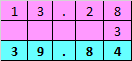Hence, the Cost of 3 ice-cream = ₹ 39.84

Correct Answer – a) ₹ 39.84

Q.7) Explanation – Money Worksheets Grade 4

Here, we have to find the cost of 1 pen when the cost of 6 pen is given
Cost of 6 pen = ₹ 144
Cost of 1 pen would be less then cost of 6 pens.
So, we have to divide cost of 6 pen, ₹ 144 by 6 to obtain the cost of 1 pen.
Cost of 1 pen = ₹ 144/6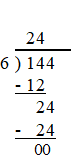Hence, the cost of 1 pen is ₹ 24.

Correct Answer – b) ₹ 24